/

### JavaScript Tutorial: Numbers and Mathematical Functions

JavaScript has only one type of number (in contrast to, e.g. Visual Basic, which differentiates between e.g. integers, floating point numbers and double precision numbers). Numbers can be written with or without decimal points and/or with or without (scientific) exponents, e.g.

var x = 4.1;    // With a decimal point

var y = 4;      // Without a decimal point

var p = 135e6   // Means 135000000

var q = 13.5e-3 // Means 0.0135

Numbers have the following properties and methods:

Properties:

 Property Description More constructor Returns object’s constructor function Here MAX_VALUE Returns largest (positive) number recognised by the browser’s JavaScript Here MIN_VALUE Returns smallest (positive) number recognised by the browser’s JavaScript Here NEGATIVE_INFINITY Represents negative infinity (i.e. if computation overflows) Here NaN Represents Not-a-Number (i.e. if computation overflows Here POSITIVE_INFINITY Represents positive infinity (i.e. if computation overflows) Here prototype Allows author to add properties and methods to an object Here

Methods:

 Method Description More isFinite() Returns true if value is a finite number, otherwise returns false Here isInteger() Returns true if value is of type Number and is an integer (within range understood as integers by the browser), otherwise returns false Here isNaN() Returns true if value is NaN, otherwise returns false Here isSafeInteger() Returns true if value is of type Number and is a safe integer, otherwise false. A safe integer is one that can be exactly represented as an IEEE-754 double precision number, i.e. is an integer in the range -(253 - 1) to (253 - 1). Here toExponential() Returns a string representing the number converted into exponential form. The optional parameter (0 to 20) represents the number of digits retained after the decimal point Here toFixed() Returns a string representing the number with a fixed number of digits after the decimal point Here toPrecision() Returns a string representing the number with a fixed number of significant digits Here toString() Returns a string corresponding to the number Here valueOf() Returns the primitive value of an object. For a number, this in effect just returns the number itself Here

The Math object

Associated with numbers is the JavaScript Math object. This allows authors to carry out some mathematical manipulations. It supports the following properties and methods:

Math object properties:

 Property Description More E Returns Euler’s constant,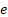Here LN2 Returns the natural logarithm of 2 Here LN10 Returns the natural logarithm of 10 Here LOG2E Returns the base-2 logarithm ofHere LOG10E Returns the base-10 logarithm ofHere PI Returns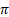Here SQRT1_2 Returns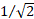Here SQRT2 Returns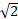Here

Math object methods:

 Method Description More abs() Returns the absolute value of a real number Here acos() Returns the (principal) arccosine of a real number Here acosh() Returns the (principal) hyperbolic arccosine of a real number Here asin() Returns the (principal) arcsine of a real number Here asinh() Returns the (principal) hyperbolic arcsine of a real number Here atan() Returns the (principal) arctangent of a real number Here atanh() Returns the (principal) hyperbolic arctangent of a real number Here atan2() Returns the arctangent of the specified x- and y-coordinates Here cbrt() Returns the cube root of a real number Here ceil() Rounds a real number towards positive infinity Here cos() Returns the cosine of a real number Here cosh() Returns the hyperbolic cosine of a real number Here exp() Returns the exponential of a real number (i.e.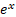) Here floor() Rounds a real number towards negative infinity Here log() Returns the natural logarithm of a positive real number Here max() Returns the maximum of a set of real numbers Here min() Returns the minimum of a set of real numbers Here pow() Returns x to the power y. Note, ^ has a different meaning in JavaScript Here random() Returns a (uniform) random number between 0 and 1 Here round() Rounds a real number to the nearest integer Here sin() Returns the sine of a real number Here sinh() Returns the hyperbolic sine of a real number Here sqrt() Returns the square root of a real (non-negative) number Here tan() Returns the tangent of a real number Here tan() Returns the hyperbolic tangent of a real number Here